Next: Motion in Uniform Fields Up: Charged Particle Motion Previous: Charged Particle Motion

# Introduction

All descriptions of plasma behaviour are based, ultimately, on the motions of the constituent particles. For the case of an unmagnetized plasma, the motions are fairly trivial, since the constituent particles move essentially in straight lines between collisions. The motions are also trivial in a magnetized plasma where the collision frequency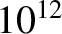greatly exceeds the gyrofrequency: in this case, the particles are scattered after executing only a small fraction of a gyro-orbit, and, therefore, still move essentially in straight lines between collisions. The situation of primary interest in this section is that of a collisionless (i.e.,), magnetized plasma, where the gyroradiusis much smaller than the typical variation length-scaleof the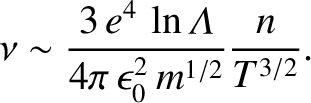andfields, and the gyroperiod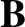is much less than the typical time-scale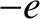on which these fields change. In such a plasma, we expect the motion of the constituent particles to consist of a rapid gyration perpendicular to magnetic field-lines, combined with free-streaming parallel to the field-lines. We are particularly interested in calculating how this motion is affected by the spatial and temporal gradients in theandfields. In general, the motion of charged particles in spatially and temporally non-uniform electromagnetic fields is extremely complicated: however, we hope to considerably simplify this motion by exploiting the assumed smallness of the parameters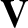and. What we are really trying to understand, in this section, is how the magnetic confinement of an essentially collisionless plasma works at an individual particle level. Note that the type of collisionless, magnetized plasma considered in this section occurs primarily in magnetic fusion and space plasma physics contexts. In fact, in the following we shall be studying methods of analysis first developed by fusion physicists, and illustrating these methods primarily by investigating problems of interest in magnetospheric physics.Next: Motion in Uniform Fields Up: Charged Particle Motion Previous: Charged Particle Motion
Richard Fitzpatrick 2011-03-31